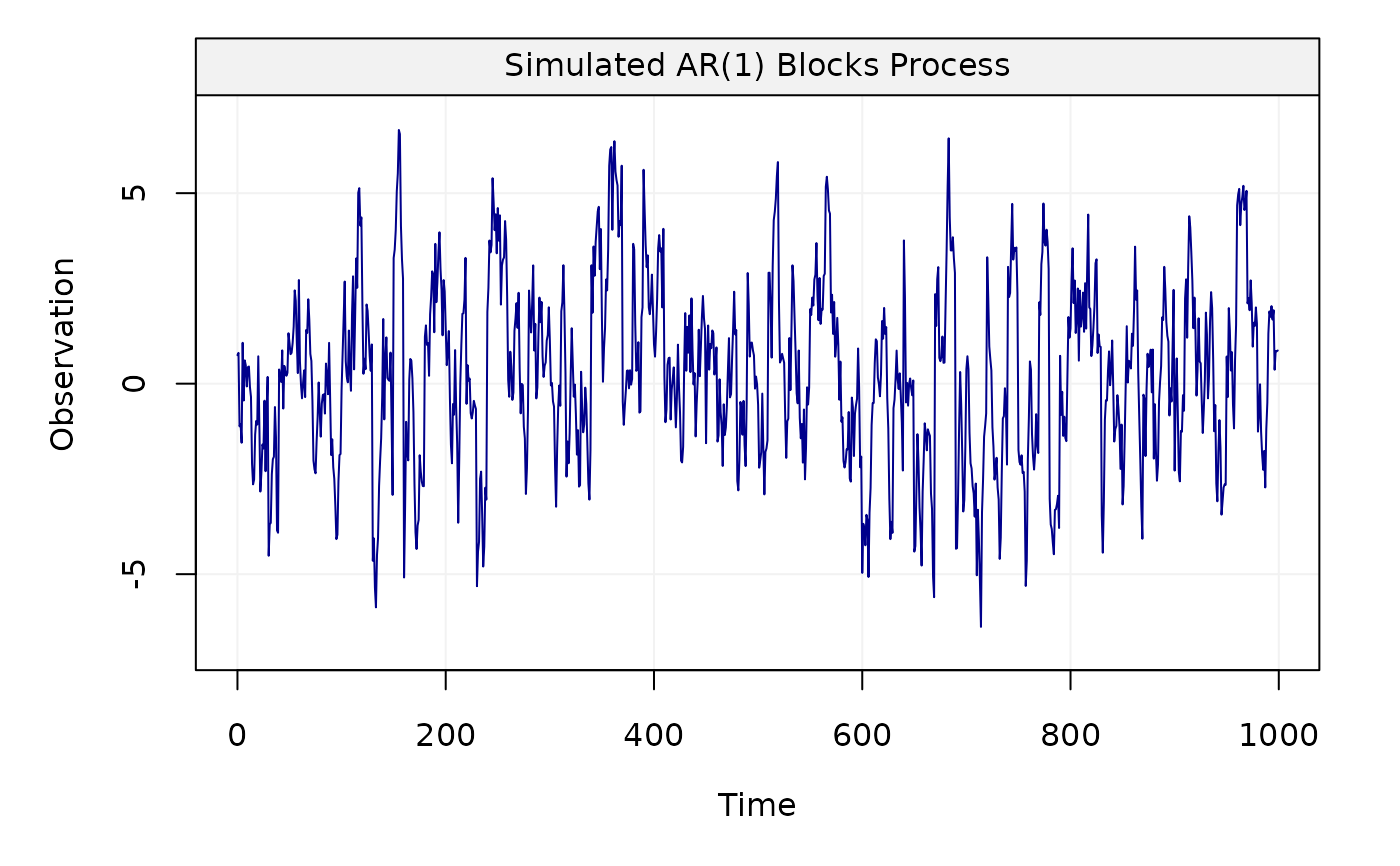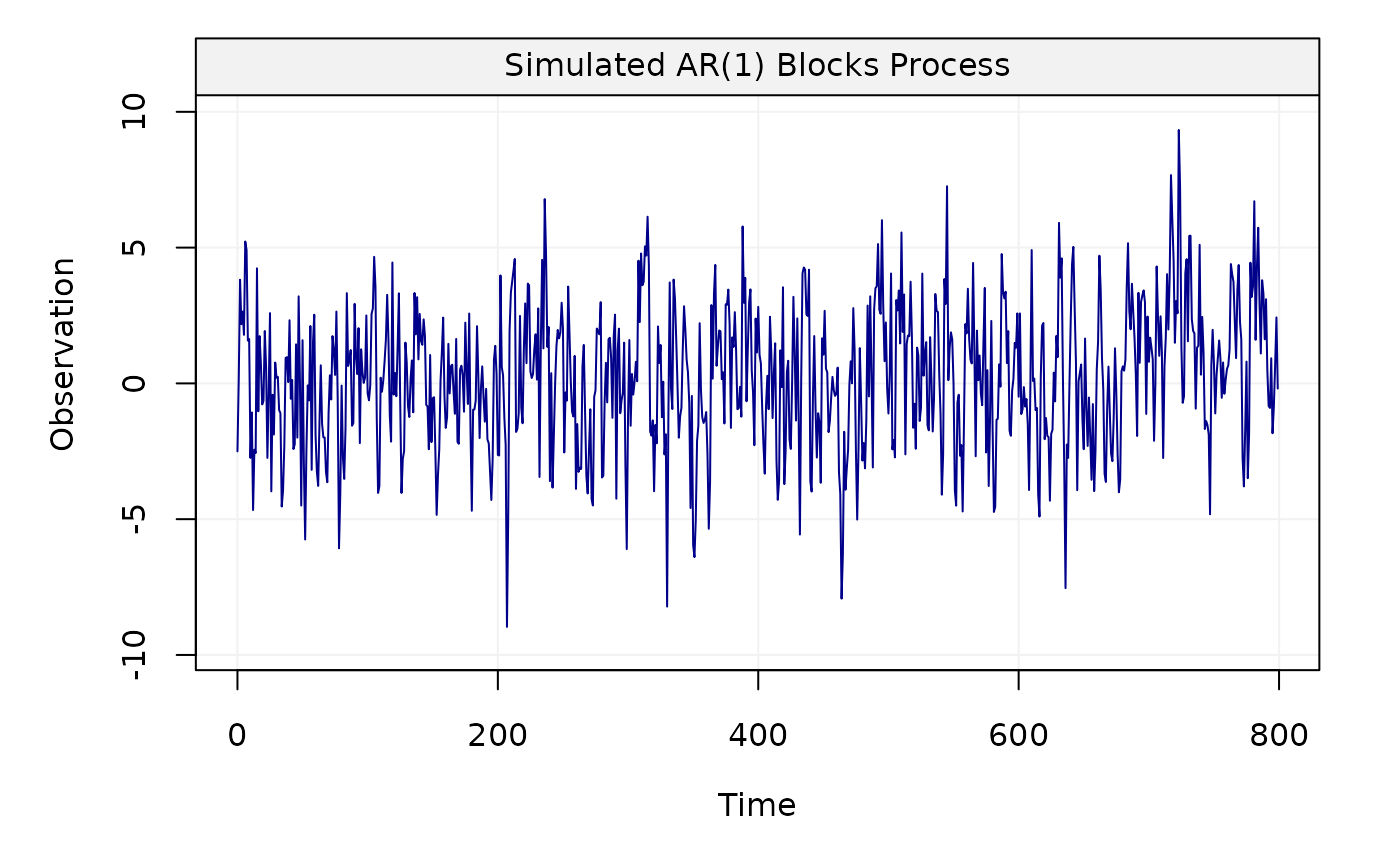This function allows us to generate a non-stationary AR(1) block process.

gen_ar1blocks(phi, sigma2, n_total, n_block, scale = 10,
title = NULL, seed = 135, ...)

## Arguments

phi

A double value for the autocorrection parameter $$\phi$$.

sigma2

A double value for the variance parameter $$\sigma ^2$$.

n_total

An integer indicating the length of the simulated AR(1) block process.

n_block

An integer indicating the length of each block of the AR(1) block process.

scale

An integer indicating the number of levels of decomposition. The default value is 10.

title

A string indicating the name of the time series data.

seed

An integer defined for simulation replication purposes.

...

## Value

A vector containing the AR(1) block process.

## Note

This function generates a non-stationary AR(1) block process whose theoretical maximum overlapping allan variance (MOAV) is different from the theoretical MOAV of a stationary AR(1) process. This difference in the value of the allan variance between stationary and non-stationary processes has been shown through the calculation of the theoretical allan variance given in "A Study of the Allan Variance for Constant-Mean Non-Stationary Processes" by Xu et al. (IEEE Signal Processing Letters, 2017), preprint available: https://arxiv.org/abs/1702.07795.

## Author

Yuming Zhang and Haotian Xu

## Examples

Xt = gen_ar1blocks(phi = 0.9, sigma2 = 1,
n_total = 1000, n_block = 10, scale = 100)
plot(Xt)Yt = gen_ar1blocks(phi = 0.5, sigma2 = 5, n_total = 800,
n_block = 20, scale = 50)
plot(Yt)